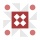Home
IT Knowledge
Inspiration
Languages
EN

# CPU Benchmark - Raspberry PI vs Banana PI - single core speed test with Fibonacci Sequence

4 points
Created by:Niac
208

In this short article, I would like to show the results of a simple CPU test made on Raspberry PI and Banana PI using Fibonacci Sequence, run on a single core.

Test result:

 Computer Name Calculations Time [s] Operating System Computer Specification Raspberry PI 2 B+ 234 Raspbian Homepage Raspberry PI 3 B+ 201 Raspbian Homepage Banana PI M3 142 Raspbian Homepage Ryzen 9 5900X 2 Windows 10 (x64) Compiler: MSVC x64 Homepage

Note: smaller calculations time means the pc is faster
(e.g. Banana PI M3 is 1.65 times faster than Raspberry PI 2 B+ on single CPU core computation)

Note: below tests were compiled with GCC (with disabled optimization).

Used test (`program.cpp` file):

``````//CPU test

#include <iostream>
#include <time.h>

int main()
{
unsigned long tmp, a = 0, b = 1;

//std::cout << a << std::endl;
//std::cout << b << std::endl;

std::cout << "Started!" << std::endl;

time_t t1 = time(NULL);

for (unsigned long i = 0uL; i < 10000uL; ++i)
{
for (unsigned long j = 0uL; j < 1000000uL; ++j)
{
tmp = b;
b = a + b;
a = tmp;

//std::cout << b << std::endl;
}
}

time_t t2 = time(NULL);
time_t dt = t2 - t1;

std::cout << "Finished!" << std::endl;

std::cout << "Result: " << b << std::endl;
std::cout << "Time: " << dt << "s" << std::endl;

return 0;
}``````

Compilation:

``````g++ -o program program.cpp

# or:

gcc -o program program.cpp``````

Running

``````chmod a+x ./program
./program``````